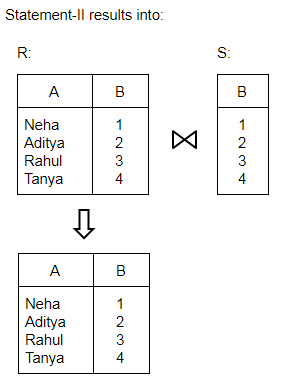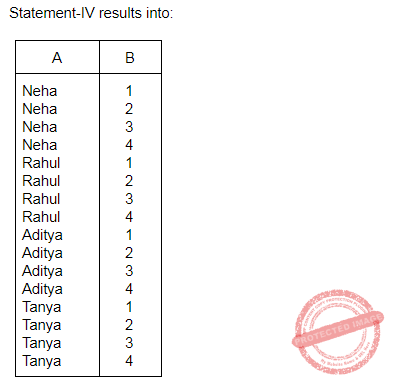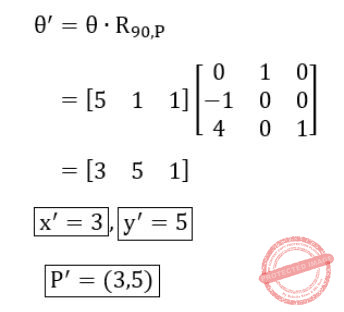## UGC NET CS 2016 July- paper-3

 Question 1
Which of the following is a sequential circuit?
 A Multiplexer B Decoder C Counter D Full adder
Digital-Logic-Design       Sequential-Circuits
Question 1 Explanation:
Combinational circuits have no memory. Combinational circuits are defined as the time independent circuits which do not depend upon previous inputs to generate any output are termed as combinational circuits.
Examples: Encoder, Decoder, Multiplexer, Demultiplexer
Sequential circuits have memory. Sequential circuits are those which are dependent on clock cycles and depends on present as well as past inputs to generate any output.
Example: Flip-flops, Counters
 Question 2
8085 microprocessor has _____ hardware interrupts.
 A 2 B 3 C 4 D 5
Computer-Organization       Microprocessor
Question 2 Explanation:Question 3
Which of the following in 8085 microprocessor performs HL = HL + DE ?
Computer-Organization       Microprocessor
Question 3 Explanation:
DAD: - Add register pair to HL register. The 16-bit contents of the specified register pair are added to the contents of the HL register and the sum is stored in the HL register. The contents of the source register pair are not altered. If the result is larger than 16 bits, the CY flag is set. No other flags are affected.
HL = HL + DE means DAD D
 Question 4
The register that stores all interrupt requests is:
 A Interrupt mask register B Interrupt service register C Interrupt request register D Status register
Computer-Organization       Interruption
Question 4 Explanation:
Status register(SR):
→ A status register is a collection of status flag bits for a processor.
→ The status register is a hardware register that contains information about the state of the processor. Individual bits are implicitly or explicitly read and/or written by the machine code instructions executing on the processor.
→ The status register lets an instruction take action contingent on the outcome of a previous instruction.
Interrupt service register(ISR):
→ Interrupt service routine(ISR) is a special block of code associated with a specific interrupt condition.
→ Interrupt handlers are initiated by hardware interrupts, software interrupt instructions, or software exceptions, and are used for implementing device drivers or transitions between protected modes of operation, such as system calls.
→ This register enables or masks interrupts from being triggered on the external pins of the Cache Controller.
→ The IMR is used to disable (Mask) or enable (Unmask) individual interrupt request inputs. This is also an 8-bit register.
Interrupt request register(IRR):
→ It stores all the interrupt inputs that are requesting service.
→ It is an 8-bit register, one bit for each interrupt request.
→ It keeps track of which interrupt inputs are asking for service. If an interrupt input is unmasked, and has an interrupt signal on it, then the corresponding bit in the IRR will be set.
→ The content of this register can be read to know the status of pending interrupts.
 Question 5
The _____ addressing mode is similar to register indirect addressing mode, except that an offset is added to the contents of the register. The offset and register are specified in the instruction.
 A Base indexed B Base indexed plus displacement C Indexed D Displacement
 Question 6
In _____ method, the word is written to the block in both the cache and main memory, in parallel.
 A Write through B Write back C Write protected D Direct mapping
Computer-Organization       Cache
Question 6 Explanation:
→ A cache memory contains copies of data stored in the main memory. When a change of data in a cache takes place (ex. a modification due to a processor write) the contents of the main memory and cache memory cells with the same address, are different. To eliminate this lack of data coherency two methods are applied:
write through, the new cache contents is written down to the main memory immediately after the write to the cache memory,
write back, the new cache contents is not written down to the main memory immediately after the change, but only when the given block of data is replaced by a new block fetched from the main memory or an upper level cache. After a data write to the cache, only state bits are changed in the modified block, indicating that the block has been modified (a dirty block).
The write back updating is more time efficient, since the block cells that were modified many times while being in the cache, are updated in the main memory only once.
 Question 7
Which of the following statements concerning Object-Oriented databases is FALSE?
 A Objects in an object-oriented database contain not only data but also methods for processing the data. B Object-oriented databases store computational instructions in the same place as the data. C Object-oriented databases are more adept at handling structured (analytical) data than relational databases. D Object-oriented databases store more types of data than relational databases and access that data faster.
Database-Management-System       OODBMS
Question 7 Explanation:
Object-oriented databases have adopted many of the concepts that were developed originally for object-oriented programming languages.
These include:
Object identity: Objects have unique identities that are independent of their attribute values and are generated by the ODMS.
Type constructors: Complex object structures can be constructed by applying in a nested manner a set of basic constructors, such as tuple, set, list, array, and bag.
Encapsulation of operations: Both the object structure and the operations that can be applied to individual objects are included in the type definitions.
Programming language compatibility: Both persistent and transient objects are handled seamlessly. Objects are made persistent by being reachable from a persistent collection (extent) or by explicit naming.
Type hierarchies and inheritance: Object types can be specified by using a type hierarchy, which allows the inheritance of both attributes and methods (operations) of previously defined types. Multiple inheritance is allowed in some models.
Extents: All persistent objects of a particular type can be stored in an extent. Extents corresponding to a type hierarchy have set/subset constraints enforced on their collections of persistent objects.
Polymorphism and operator overloading: Operations and method names can be overloaded to apply to different object types with different implementations.
 Question 8
In distributed databases, location transparency allows for database users, programmers and administrators to treat the data as if it is at one location. A SQL query with location transparency needs to specify:
 A Inheritances B Fragments C Locations D Local formats
Database-Management-System       Databases
Question 8 Explanation:
In distributed databases, location transparency allows for database users, programmers and administrators to treat the data as if it is at one location. A SQL query with location transparency needs to specify fragments.
 Question 9
Consider the relations R(A, B) and S(B, C) and the following four relational algebra queries over R and S:

I. ΠA, B (R ⨝ S)

II. R ⨝ ΠB(S)

III. R ∩ (ΠA(R) × ΠB(S))

IV. ΠA, R.B (R × S)

where R⋅B refers to the column B in table R.

One can determine that:

 A I, III and IV are the same query. B II, III and IV are the same query. C I, II and IV are the same query. D I, II and III are the same query.
Database-Management-System       Relational-Algebra
Question 9 Explanation:Question 10
Which of the following statements is TRUE?

D1 : The decomposition of the schema R(A, B, C) into R1(A, B) and R2 (A, C) is always lossless.

D2 : The decomposition of the schema R(A, B, C, D, E) having AD → B, C → DE, B → AE and AE → C, into R1 (A, B, D) and R2 (A, C, D, E) is lossless.

 A Both D1 and D2 B Neither D1 nor D2 C Only D1 D Only D2
Database-Management-System       Functional-Dependency
Question 10 Explanation:
Statement(1) is incorrect because functional dependencies of relation R are not given, so we can't say whether the decomposition of R into R1 and R2 is lossless or not.Question 11
Consider the following ORACLE relations :

R (A, B, C) = {<1, 2, 3>, <1, 2, 0>, <1, 3, 1>, <6, 2, 3>, <1, 4, 2>, <3, 1, 4> }

S (B, C, D) = {<2, 3, 7>, <1, 4, 5>, <1, 2, 3>, <2, 3, 4>, <3, 1, 4>}.

Consider the following two SQL queries SQ1 and SQ2 :

SQ1 : SELECT R⋅B, AVG (S⋅B) FROM R, S WHERE R⋅A = S⋅C AND S⋅D < 7 GROUP BY R⋅B;

SQ2 : SELECT DISTINCT S⋅B, MIN (S⋅C) FROM S GROUP BY S⋅B HAVING COUNT (DISTINCT S⋅D) > 1;

If M is the number of tuples returned by SQ1 and N is the number of tuples returned by SQ2 then

 A M = 4, N = 2 B M = 5, N = 3 C M = 2, N = 2 D M = 3, N = 3
Database-Management-System       SQL
Question 11 Explanation:Question 12
Semi-join strategies are techniques for query processing in distributed database system. Which of the following is a semi-join technique ?
 A Only the joining attributes are sent from one site to another and then all of the rows are returned. B All of the attributes are sent from one site to another and then only the required rows are returned. C Only the joining attributes are sent from one site to another and then only the required rows are returned. D All of the attributes are sent from one site to another and then only the required rows are returned.
Database-Management-System       Relational-Algebra
Question 12 Explanation:
A semi-join returns one copy of each row in the first table for which at least one match is found. Semi-join is like EXCEPT command in SQL.
 Question 13
Consider the Breshenham’s circle generation algorithm for plotting a circle with centre (0, 0) and radius ‘r’ units in first quadrant. If the current point is (xi , yi ) and decision parameter is pi then what will be the next point (xi + 1, yi + 1 + 1) and updated decision parameter pi + 1 for pi ≥ 0?
 A xi + 1 = xi+ 1 yi + 1 = yi pi + 1 = pi + 4xi + 6 B xi + 1 = xi + 1 yi + 1 = yi - 1 pi + 1 = pi + 4(xi - yi) + 10 C xi + 1 = xi yi + 1 = yi - 1 pi + 1 = pi + 4(xi - yi) + 6 D xi + 1 = xi - 1 yi + 1 = yi pi + 1 = pi + 4(xi - yi) + 10
Computer-Graphics       Bresenham’s-Algorithm
Question 13 Explanation:
Write the steps required to scan - convert a circle using Bresenham’s algorithm.
Set the initial values of the variables: (h, k) = coordinates of circle center; x=0; y=circle radius r and d = 3 - 2r.
Test to determine whether the entire circle has been scan-converted. If x>y, stop.
Plot the eight points, found by symmetry with respect to the center (h, k), at the current (x, y) coordinates:
Plot(x+h, y+k) Plot(-x+h, -y+k)
Plot(y+h, x+k) Plot(-y+h, -x+k)
Plot(-y+h, x+k) Plot(y+h, -x+k)
Plot(-x+h, y+k) Plot(x+h, -y+k)
Compute the location of the next pixel. If d<0, then d=d+4x+6 and x=x+1. If d≥0, then d=d+4(x-y)+10, x=x+1 and y=y-1.
Go to step 2.
 Question 14
A point P(5, 1) is rotated by 90° about a pivot point (2, 2). What is the coordinate of new transformed point P′ ?
 A (3, 5) B (5, 3) C (2, 4) D (1, 5)
Computer-Graphics       Transformations
Question 14 Explanation:
Rotation around a pivot point (2, 2) can be represented as:
(h, k) = (2, 2)Question 15
Let R be the rectangular window against which the lines are to be clipped using 2D Sutherland-Cohen line clipping algorithm. The rectangular window has a lower left-hand corner at (– 5, 1) and upper right-hand corner at (3, 7). Consider the following three lines for clipping with the given endpoint coordinates:
Line AB : A (– 6, 2) and B (–1, 8)
Line CD : C (– 1, 5) and D (4, 8)
Line EF : E (–2, 3) and F (1, 2)
Which of the following line(s) is/are candidate for clipping?
 A AB B CD C EF D AB and CD
Computer-Graphics       Sutherland-Cohen-algorithm
 Question 16
In perspective projection, if a line segment joining a point which lies in front of the viewer to a point in back of the viewer is projected to a broken line of infinite extent. This is known as _______.
 A View confusion B Vanishing point C Topological distortion D Perspective foreshortening
Computer-Graphics       Topological-Distortion
 Question 17
Let us consider that the original point is (x, y) and new transformed point is (x′, y′). Further, Shx and Shy are shearing factors in the x and y directions. If we perform the y-direction shear relative to x = xref then the transformed point is given by _______.
 A x′ = x + Shx ⋅ (y – yref) y′ = y B x′ = x y′ = y ⋅ Shx C x′ = x y′ = Shy (x – xref) + y D x′ = Shy ⋅ y y′ = y ⋅ (x – xref)
Computer-Graphics       Transformations
Question 17 Explanation:Question 18
Which of the following statement(s) is/are correct with reference to curve generation?
I. Hermite curves are generated using the concepts of interpolation.
II. Bezier curves are generated using the concepts of approximation.
III. The Bezier curve lies entirely within the convex hull of its control points.
IV. The degree of Bezier curve does not depend on the number of control points.
 A I, II and IV only B II and III only C I and II only D I, II and III only
Computer-Graphics       Bezier -Curves
There are 18 questions to complete.

Register Now